# One Number As A Percentage Of Another Worksheet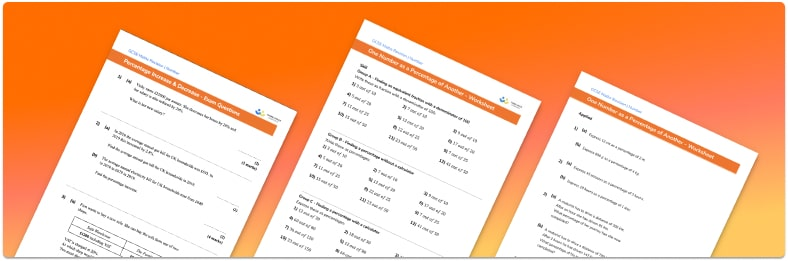Help your students prepare for their Maths GCSE with this free one number as a percentage of another worksheet of 43 questions and answers

• Section 1 of the one number as a percentage of another worksheet contains 36 skills based one number as a percentage of another questions, in 3 groups to support differentiation
• Section 2 contains 4 applied one number as a percentage of another questions with a mix of worded problems and deeper problem solving questions
• Section 3 contains 3 foundation and higher level GCSE exam style one number as a percentage of another questions
• Answers and a mark scheme for all 43 questions
• Follows variation theory with plenty of opportunities for students to work independently at their own level
• All questions created by fully qualified expert secondary maths teachers
• Suitable for GCSE maths revision for AQA, OCR and Edexcel exam boards
• To receive this resource and regular emails with more free resources, blog posts and other Third Space updates, enter your email address and click below.

• This field is for validation purposes and should be left unchanged.

You can unsubscribe at any time (each email we send will contain an easy way to unsubscribe). To find out more about how we use your data, see our privacy policy.

### One number as a percentage of another at a glance

It can often be useful to write one number as a percentage of another. Common examples of when we might write one number as a percentage of another include for test scores, when talking about attendance to school or to an event or when working out discounts.

There are two ways to write one number as a percentage of another. The first is to use equivalent fractions by finding a fraction with a denominator of 100. The numerator is then the percentage because percent means out of 100. For example, 7 over 10 is equal to 70 over 100 which is equal to 70%.

The second way is to use a calculator. We can divide the numerator by the denominator to find the decimal and then multiply by 100 to get the percentage. For example to write 31 over 40 as a percentage we can calculate 31 ÷ 40 x 100 = 77.5%.

Looking forward, students can then progress to additional number worksheets, for example aor aFor more teaching and learning support on Number our GCSE maths lessons provide step by step support for all GCSE maths concepts.

## Related worksheets

Percentage Multiplier Worksheet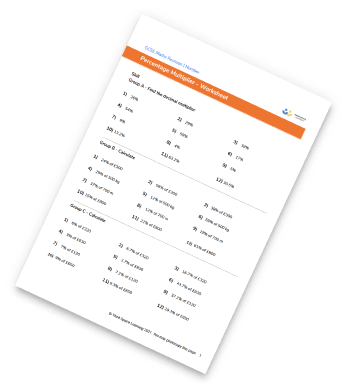Reverse Percentages Worksheet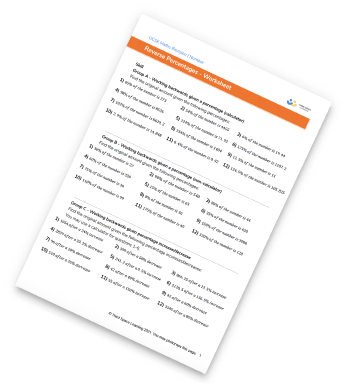Percentage Increase And Decrease Worksheet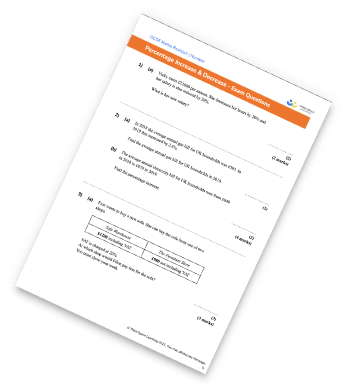Percentage Worksheet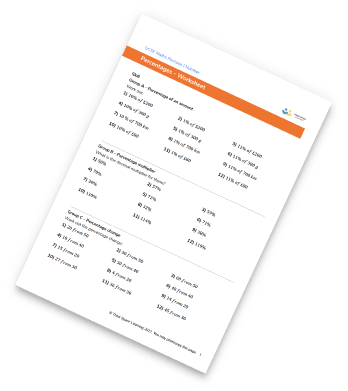## Do you have KS4 students who need more focused attention to succeed at GCSE?There will be students in your class who require individual attention to help them succeed in their maths GCSEs. In a class of 30, it’s not always easy to provide.

Help your students feel confident with exam-style questions and the strategies they’ll need to answer them correctly with our dedicated GCSE maths revision programme.

Lessons are selected to provide support where each student needs it most, and specially-trained GCSE maths tutors adapt the pitch and pace of each lesson. This ensures a personalised revision programme that raises grades and boosts confidence.

Find out more